# At what temperature will the aluminum and steel segments in Prob. 2.86 have stresses of equal magnitude after the 50-kip force is applied?

Question-AnswerCategory: Strength of MaterialsAt what temperature will the aluminum and steel segments in Prob. 2.86 have stresses of equal magnitude after the 50-kip force is applied?

At what temperature will the aluminum and steel segments in Prob. 2.86 have stresses of equal magnitude after the 50-kip force is applied?Step: 1

Draw the freebody diagram of composite bar .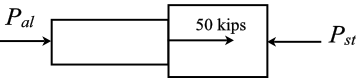Step: 2

Determine the stress in each part when stress is equal.
Consider forces along horizontal direction.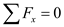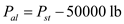Here,is force in steel segment and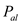is force in aluminum segment.
Substituteforandfor.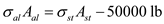Step: 3

Here,is stress in steel part,is stress in aluminum part,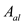is cross section n area of aluminum and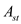is cross section n area of steel.

Step: 4

Substitute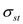for,forandfor.Therefore,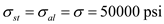Step: 5

Draw the displacement diagram .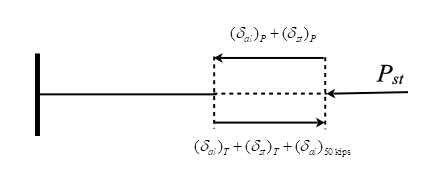Step: 6

Calculate the at what temperature will aluminum and steel segment have stress of equal magnitude after the loadis applied by using the compatibility equation (relaxing the right support).
Compatibility equation isHere,is length of aluminum segment,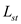is length of steel segment,is coefficient of thermal expansion of aluminum,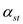is coefficient of thermal expansion of rod ,is temperature change,is cross section area of aluminum segment,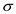is stress in each segment,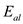is Young’s modulus of aluminum and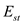is Young’s modulus of steel.
Substitutefor,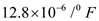for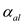,for,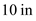for,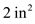for,for,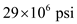for,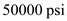forand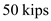for.The final temperature becomes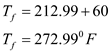Therefore, the at what temperature will aluminum and steel segment have stress of equal magnitude after the loadis applied is